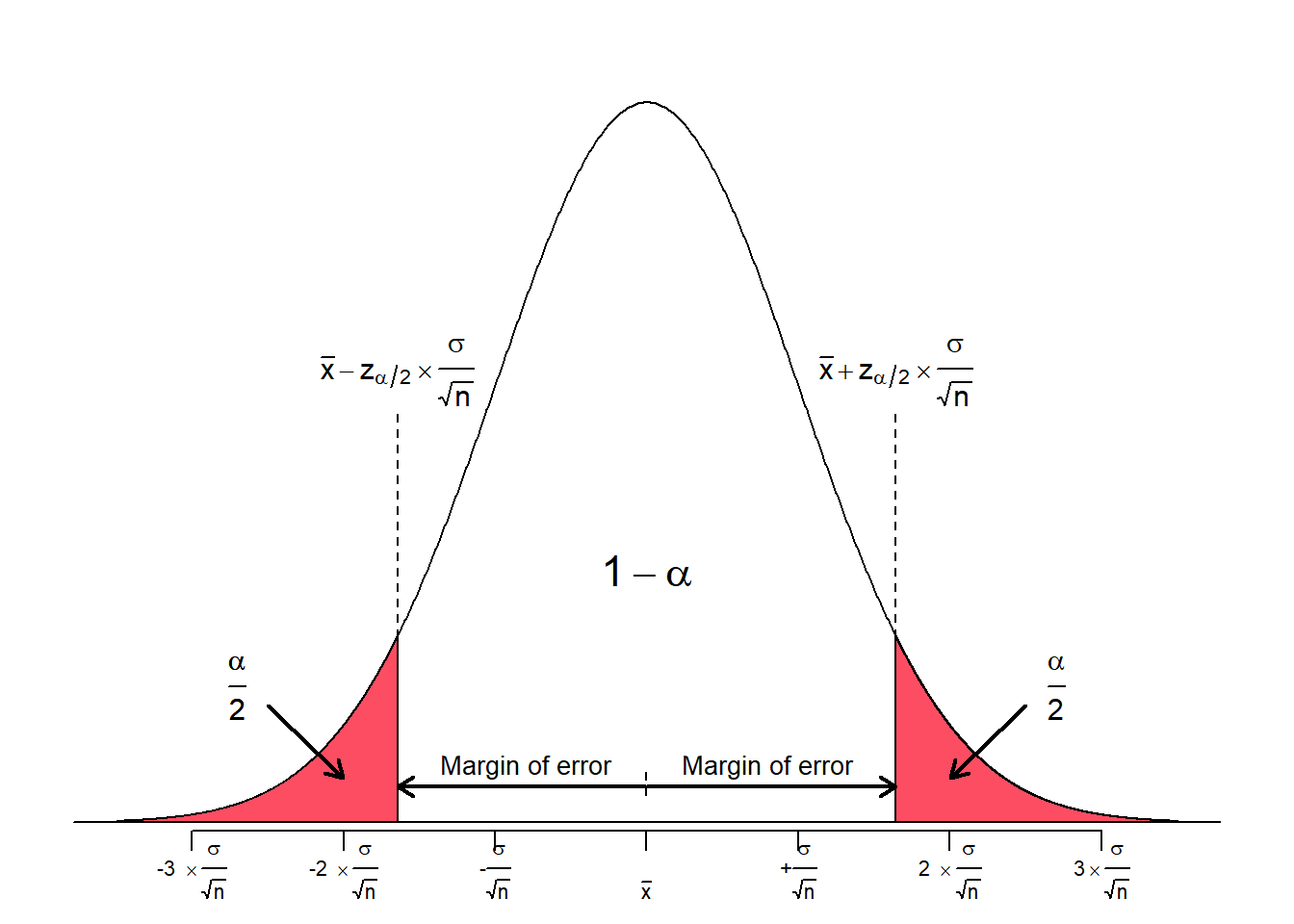Instead of assigning a single value to a population parameter, an interval estimation gives a probabilistic statement, relating the given interval to the probability that this interval actually contains the true (unknown) population parameter.

The level of confidence is chosen a priori and thus depends on the users preferences. It is denoted by

$100(1-\alpha) \%$

Although any value of confidence level can be chosen, the most common values are 90 %, 95 % and 99 %. When expressed as probability, the confidence level is called the confidence coefficient and is denoted by ($$1 - \alpha$$). Most common confidence coefficients are 0.90, 0.95 and 0.99, respectively.

A $$100(1-\alpha)\%$$ confidence interval is an interval estimate around a population parameter $$\theta$$ (here, the Greek letter $$\theta$$ (theta) is a placeholder for any population parameter of interest, such as the mean $$\mu$$ or the standard deviation $$\sigma$$, among others) that, under repeated random samples of size $$N$$, is expected to include $$\theta$$’s true value $$100(1-\alpha)\%$$ of the time (Lovric 2010).

The actual number added to and subtracted from the point estimate is called the margin of error.

$CI: \text{Point estimate} \pm \text{Margin of error}$

The margin of error constitutes of two entities. First, the so called critical value and second, a measure of variability of the sampling distribution. The critical value is a numerical value that corresponds to the a priori set level of confidence. It is denoted as $$z^*$$. The relation to the level of confidence is made explicit by the subscript $$z^*_{\alpha/2}$$.

Note: The confidence interval has a lower and an upper limit. Consequently, $$\alpha$$ is divided by 2 as the area under the curve beyond those limits corresponds to $$\frac{\alpha}{2} \times 2 = \alpha$$.

The measure of variability is the standard error, denoted as $$\frac{\sigma}{\sqrt{n}}$$, if $$\sigma$$ is known. If $$\sigma$$ is not known, the sample standard error given by $$\frac{s}{\sqrt{n}}$$, where $$s$$ is the standard deviation of the sample, may be chosen instead.

Thus, the margin of error (ME) is expressed as

$ME = z^*_{\alpha/2} \times \frac{\sigma}{\sqrt{n}}$

Let us look at a figure for better comprehension:Accordingly, the full equation for the confidence interval is given by

$CI: \text{Point estimate} \pm z^*_{\alpha/2} \times \frac{\sigma}{\sqrt{n}}$

In order to get the corresponding value for $$z^*_{\alpha/2}$$ one may look it up in a table or make use of the qnorm() function in R. Make sure to apply the lower.tail argument in the qnorm() function properly.

Let us construct some confidence intervals for practice:

Confidence level of 90 % ($$\alpha = 0.1$$)

lower_90 <- qnorm(0.05, lower.tail = TRUE)
upper_90 <- qnorm(0.05, lower.tail = FALSE)

paste(
"The lower and upper limits of the interval that covers an area of 90% around the mean are given by z-scores of",
round(lower_90, 2), "and", round(upper_90, 2), "respectively."
)
##  "The lower and upper limits of the interval that covers an area of 90% around the mean are given by z-scores of -1.64 and 1.64 respectively."

For a confidence level of 90 % ($$\alpha = 0.1$$) the equation from above evaluates to

$CI_{90\%}: \text{Point estimate} \pm 1.64 \times \frac{\sigma}{\sqrt{n}}$

Confidence level of 95 % ($$\alpha = 0.05$$)

lower_95 <- qnorm(0.025, lower.tail = TRUE)
upper_95 <- qnorm(0.025, lower.tail = FALSE)

paste(
"The lower and upper limits of the interval that covers an area of 95% around the mean are given by z-scores of",
round(lower_95, 2), "and", round(upper_95, 2), "respectively."
)
##  "The lower and upper limits of the interval that covers an area of 95% around the mean are given by z-scores of -1.96 and 1.96 respectively."

For a confidence level of 95 % ($$\alpha = 0.05$$) the equation from above evaluates to

$CI_{95\%}: \text{Point estimate} \pm 1.96 \times \frac{\sigma}{\sqrt{n}}$

Confidence level of 99 % ($$\alpha = 0.01$$)

lower_99 <- qnorm(0.005, lower.tail = TRUE)
upper_99 <- qnorm(0.005, lower.tail = FALSE)

paste(
"The lower and upper limits of the interval that covers an area of 95% around the mean are given by z-scores of",
round(lower_99, 2), "and", round(upper_99, 2), "respectively."
)
##  "The lower and upper limits of the interval that covers an area of 95% around the mean are given by z-scores of -2.58 and 2.58 respectively."

For a confidence level of 99 % ($$\alpha = 0.01$$) the equation from above evaluates to $CI_{99\%}: \text{Point estimate} \pm 2.58 \times \frac{\sigma}{\sqrt{n}}$

Citation

The E-Learning project SOGA-R was developed at the Department of Earth Sciences by Kai Hartmann, Joachim Krois and Annette Rudolph. You can reach us via mail by soga[at]zedat.fu-berlin.de.You may use this project freely under the Creative Commons Attribution-ShareAlike 4.0 International License.

Please cite as follow: Hartmann, K., Krois, J., Rudolph, A. (2023): Statistics and Geodata Analysis using R (SOGA-R). Department of Earth Sciences, Freie Universitaet Berlin.